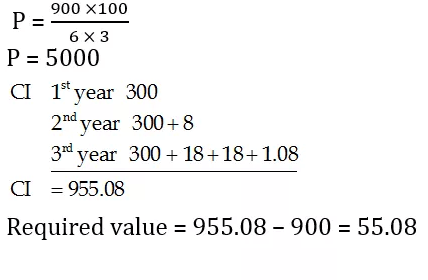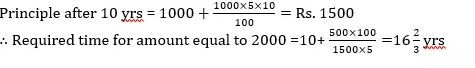Quant Quiz for SBI PO PRE & LIC AAO 2019 |29th April 2019

Quant Quiz for SBI PO PRE & LIC AAO 2019

Reasoning Quiz to improve your Quantitative Aptitude for SBI Po & SBI clerk exam Reasoning, IBPS PO Reasoning , IBPS Clerk Reasoning , IBPS RRB Reasoning, LIC AAO ,  and other competitive exams.

1. What sum will give Rs. 244 as the difference between simple interest and compound interest at 10% in 1.5 years compounded half yearly ?

A) Rs. 40,000

B) Rs. 36,000

C)  Rs. 32,000

D) Rs. 28,000

E) None of these

Q1. Ans(C)Q2. Meenu had a certain sum of money. 5/11 th of the total money she invested in Scheme X for 6 years and rest of the money she invested in Scheme Y for 2 years. Scheme X offers simple interest at rate of 18% p.a. and Scheme Y offers compound interest (compounded annually) at a rate of 20% p.a. If the interest received from Scheme X is Rs 1518 more than the interest received from Scheme Y, what was the sum invested by her in Scheme X?

A) Rs 2640

B) Rs 2750

C) Rs 2500

D) Rs 2850

E)  Rs 2550

Q2. Ans(B)Q3. Data Ram lends equal sum of money at the same rate of interest to A and B. The money lends to A becomes twice of the original amount in just four years at simple interest. While Data Ram lends to B for the first two years at compound interest and for the rest two years at simple interest. If the difference between the amount of A and B after 4 years is Rs. 2750. What is the amount of money that Data Ram lends to each one?

A) Rs. 40000

B) Rs. 6000

C) Rs. 8000

D) Rs. 80000

E) none of these

Q3. Ans(C)Q4. Rs. 6100 was partly invested in Scheme A at 10% p.a. compound interest (compounded annually) for 2 years and partly in Scheme B at 10% p.a. simple interest for 4 years. Both the schemes give equal interests. How much was invested in Scheme A ?

A) Rs. 3750

B) Rs. 4500

C) Rs. 4000

D) Rs. 3250

E)  Rs. 5000

Q4. Ans(C)Q5. Amit puts some amount in bank and obtained an interest of Rs. 15 at the end of one year. He added Rs. 85 to his amount and put the whole amount again in the bank for another year. At the end of the 2nd year, Amit got Rs. 420 as total (interest + principal). What amount did he put in the beginning and what is the rate of interest offered by the bank if the minimum amount that can be deposited in the bank is Rs. 75 ?

A) 250, 5%

B)  300, 5%

C) 200, 8%

D) 350, 3.5%

E) None of these

Q5. Ans(B)Q6.  Rakesh borrows Rs. 3500 from a bank at SI. After three years he paid Rs. 1500 to the bank and at the end of 5 years from the date of borrowing he paid Rs. 2725 to the bank to settle the account. Find the rate of interest.

A) 10%

B) 5%

C) 2.5%

D) 3.5%

E) None of these

Q6. Ans(B)Q7. Ashok borrowed some money at simple interest at the rate of 6 per cent per annul for the first two years, at the rate of 9 per cent per annul for the next three years and at the rate of 14% per cent per annul for the period beyond five years. If he pays a total interest of Rs. 11400 at the end of 9 years how much money did he borrow ?

(a) Rs. 16,000

(b) Rs. 14,000

(c) Rs. 18,000

(d) Rs. 12,000

(e) None of these

Q7. Ans(d)Q8. On Rs 3500 invested at a simple interest rate 7 per cent per annum, Rs 500 is obtained as interest in certain years, In order to earn Rs 800 as interest on Rs 4900 in the same number of years, what should be the rate of simple interest?

(a) 9%

(b) 10%

(c) 12%

(d) 8%

(e) None of these

Q8. Ans(d)Q9. A person invested a certain amount at simple interest at the rate of 6 per cent per annul earning Rs. 900 as an interest at the end of three years. Had the interest been compounded every year, how much more interest would he have earned on the same amount with the same interest rate after three years ?

(a) Rs. 38.13

(b) Rs. 25.33

(c) Rs. 55.08

(d) Rs. 35.30

(e) None of these

Q9. Ans(c)Q10. If Rs.1000 be invested at interest rate of 5% and the interest be added to the principle every 10 years, then In how many years  it will amount to Rs. 2000 ?Q10. Ans(a)You Can Read This Also:

Best E-books for LIC AAO 2019 : Get PDF here

ambitiousbaba.com need your support to Grow

I challenge you will get Best Content in Our PDFs with Detail solutions and Latest Pattern

Memory Based Puzzle E-book | 2016-19 Exams Covered

Get PDF here

Caselet Data Interpretation 200 Questions

Get PDF here
Puzzle & Seating Arrangement E-Book for BANK PO MAINS (Vol-1)

Get PDF here

ARITHMETIC DATA INTERPRETATION 2019 E-book

Get PDF here
The Banking Awareness 500 MCQs E-book| Bilingual (Hindi + English)

Get PDF here

High Level DATA INTERPRETATION Practice E-BOOK

Get PDF here